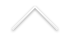Diophantine geometry consists in finding integral solutions of integral polynomial equations in many variables. It dates back to the greeks, as Pythagoras theorem recalls us. And some conjectures have been resisted quite a long period of time, like Fermat`s last theorem, about 300 years. In fact, its proof is an incarnation of the power of the successor of diophantine geometry, the arithmetic geometry. To produce solutions mentioned above, it is more interesting to understand their arithmetic complexity, measured by the height, as well as its linear incarnations through Galois representations. The fact that Fermat's last theorem is true is less interesting than the fact that it is implied by the Shimura-Tanyiama conjecture proved by Wiles, Taylor et at. This conjecture lies deep in the building of the arithmetic of elliptic curves during the second half of the twentieth century. It also involves an ubiquitous notion in mathematics, i.e., that of deformation whose origin is algebraic geometry, but is extended from Galois representations to physics.

Researchers:

• Amilcar Pacheco
• Luciane Quoos Conte
• Nicolas Puignau